Next: Multi-component systems Up: Conservation of momentum Previous: Introduction

## Two-component systems

The simplest imaginable multi-component dynamical system consists of two point mass objects which are both constrained to move along the same straight-line. See Fig. 45. Let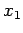be the displacement of the first object, whose mass is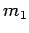. Likewise, let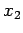be the displacement of the second object, whose mass is. Suppose that the first object exerts a force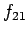on the second object, whereas the second object exerts a force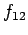on the first. From Newton's third law of motion, we have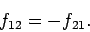(180)

Suppose, finally, that the first object is subject to an external force (i.e., a force which originates outside the system)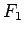, whilst the second object is subject to an external force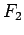.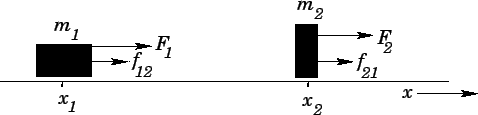Applying Newton's second law of motion to each object in turn, we obtain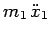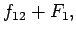(181)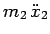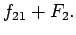(182)

Here,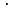is a convenient shorthand for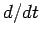. Likewise,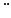means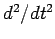.

At this point, it is helpful to introduce the concept of the centre of mass. The centre of mass is an imaginary point whose displacement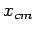is defined to be the mass weighted average of the displacements of the two objects which constitute the system. In other words,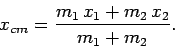(183)

Thus, if the two masses are equal then the centre of mass lies half way between them; if the second mass is three times larger than the first then the centre of mass lies three-quarters of the way along the line linking the first and second masses, respectively; if the second mass is much larger than the first then the centre of mass is almost coincident with the second mass; and so on.

Summing Eqs. (181) and (182), and then making use of Eqs. (180) and (183), we obtain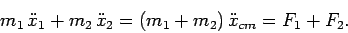(184)

Note that the internal forces,and, have canceled out. The physical significance of this equation becomes clearer if we write it in the following form: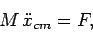(185)

where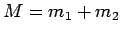is the total mass of the system, and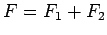is the net external force acting on the system. Thus, the motion of the centre of mass is equivalent to that which would occur if all the mass contained in the system were collected at the centre of mass, and this conglomerate mass were then acted upon by the net external force. In general, this suggests that the motion of the centre of mass is simpler than the motions of the component masses,and. This is particularly the case if the internal forces,and, are complicated in nature.

Suppose that there are no external forces acting on the system (i.e.,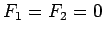), or, equivalently, suppose that the sum of all the external forces is zero (i.e.,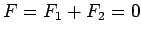). In this case, according to Eq. (185), the motion of the centre of mass is governed by Newton's first law of motion: i.e., it consists of uniform motion in a straight-line. Hence, in the absence of a net external force, the motion of the centre of mass is almost certainly far simpler than that of the component masses.

Now, the velocity of the centre of mass is written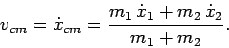(186)

We have seen that in the absence of external forces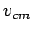is a constant of the motion (i.e., the centre of mass does not accelerate). It follows that, in this case,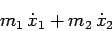(187)

is also a constant of the motion. Recall, however, from Sect. 4.3, that momentum is defined as the product of mass and velocity. Hence, the momentum of the first mass is written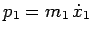, whereas the momentum of the second mass takes the form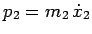. It follows that the above expression corresponds to the total momentum of the system: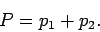(188)

Thus, the total momentum is a conserved quantity--provided there is no net external force acting on the system. This is true irrespective of the nature of the internal forces. More generally, Eq. (185) can be written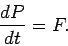(189)

In other words, the time derivative of the total momentum is equal to the net external force acting on the system--this is just Newton's second law of motion applied to the system as a whole.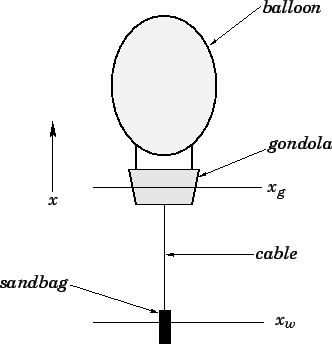Let us now try to apply some of the concepts discussed above. Consider the simple two-component system shown in Fig. 46. A gondola of masshangs from a hot-air balloon whose mass is negligible compared to that of the gondola. A sandbag of massis suspended from the gondola by means of a light inextensible cable. The system is in equilibrium. Suppose, for the sake of consistency with our other examples, that the-axis runs vertically upwards. Let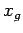be the height of the gondola, and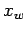the height of the sandbag. Suppose that the upper end of the cable is attached to a winch inside the gondola, and that this winch is used to slowly shorten the cable, so that the sandbag is lifted upwards a distance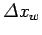. The question is this: does the height of the gondola also change as the cable is reeled in? If so, by how much?

Let us identify all of the forces acting on the system shown in Fig. 46. The internal forces are the upward force exerted by the gondola on the sandbag, and the downward force exerted by the sandbag on the gondola. These forces are transmitted via the cable, and are equal and opposite (by Newton's third law of motion). The external forces are the net downward force due to the combined weight of the gondola and the sandbag, and the upward force due to the buoyancy of the balloon. Since the system is in equilibrium, these forces are equal and opposite (it is assumed that the cable is reeled in sufficiently slowly that the equilibrium is not upset). Hence, there is zero net external force acting on the system. It follows, from the previous discussion, that the centre of mass of the system is subject to Newton's first law. In particular, since the centre of mass is clearly stationary before the winch is turned on, it must remain stationary both during and after the period in which the winch is operated. Hence, the height of the centre of mass,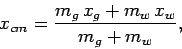(190)

is a conserved quantity.

Suppose that the operation of the winch causes the height of the sandbag to change by, and that of the gondola to simultaneously change by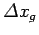. Ifis a conserved quantity, then we must have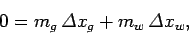(191)

or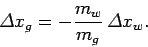(192)

Thus, if the winch is used to raise the sandbag a distancethen the gondola is simultaneously pulled downwards a distance. It is clear that we could use a suspended sandbag as a mechanism for adjusting a hot-air balloon's altitude: the balloon descends as the sandbag is raised, and ascends as it is lowered.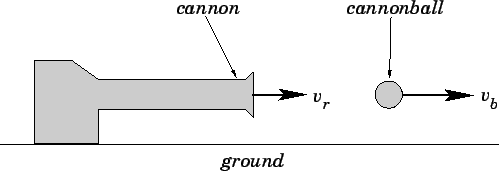Our next example is pictured in Fig. 47. Suppose that a cannon of mass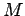propels a cannonball of masshorizontally with velocity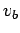. What is the recoil velocity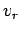of the cannon? Let us first identify all of the forces acting on the system. The internal forces are the force exerted by the cannon on the cannonball, as the cannon is fired, and the equal and opposite force exerted by the cannonball on the cannon. These forces are extremely large, but only last for a short instance in time: in physics, we call these impulsive forces. There are no external forces acting in the horizontal direction (which is the only direction that we are considering in this example). It follows that the total (horizontal) momentum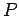of the system is a conserved quantity. Prior to the firing of the cannon, the total momentum is zero (since momentum is mass times velocity, and nothing is initially moving). After the cannon is fired, the total momentum of the system takes the form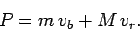(193)

Sinceis a conserved quantity, we can set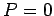. Hence,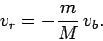(194)

Thus, the recoil velocity of the cannon is in the opposite direction to the velocity of the cannonball (hence, the minus sign in the above equation), and is of magnitude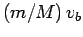. Of course, if the cannon is far more massive that the cannonball (i.e.,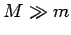), which is usually the case, then the recoil velocity of the cannon is far smaller in magnitude than the velocity of the cannonball. Note, however, that the momentum of the cannon is equal in magnitude to that of the cannonball. It follows that it takes the same effort (i.e., force applied for a certain period of time) to slow down and stop the cannon as it does to slow down and stop the cannonball.Next: Multi-component systems Up: Conservation of momentum Previous: Introduction
Richard Fitzpatrick 2006-02-02